# Python Tutorial

We will review several examples using the Python interface that demonstrate the process of computing fields, transmittance/reflectance spectra, and resonant modes. The examples are mainly 1d or 2d simulations, simply because they are quicker than 3d and they illustrate most of the essential features. For more advanced functionality involving 3d simulations with a focus on technology applications, see the Simpetus projects page.

## The Meep Library

Meep simulations are Python scripts which involve specifying the device geometry, materials, current sources, monitor fields, and everything else necessary to set up a calculation. A Python script provides the flexibility to customize the simulation for practically any application particularly those involving parameter sweeps and optimization. Python libraries such as NumPy, SciPy, and Matplotlib can be used to augment the simulation functionality and will also be demonstrated. Much of the functionality of the low-level C++ interface has been abstracted in Python which means that you don't need to be an experienced programmer to set up simulations. Reasonable defaults are available where necessary.

Executing Meep simulations is normally done at the Unix command line as follows:

 unix% python foo.py >& foo.out


which reads the Python script foo.py and executes it, saving the output to the file foo.out. If you want to set up simulations in interactive mode where you can type commands and see the results immediately, you will need to use either IPython via a shell terminal or a Jupyter notebook via a browser. If you use one of these approaches, you can paste in the commands from the tutorial as you follow along and see what they do.

## Fields in a Waveguide

For our first example, let's examine the field pattern excited by a localized CW source in a waveguide — first straight, then bent. The waveguide will have frequency-independent $\varepsilon=12$ and width 1 μm. The unit length in this example is 1 μm. See also Units.

### A Straight Waveguide

The simulation script is in examples/straight-waveguide.py. The notebook is examples/straight-waveguide.ipynb.

The first thing to do always is to load the Meep library:

import meep as mp


We can begin specifying each of the simulation objects starting with the computational cell. We're going to put a source at one end and watch the fields propagate down the waveguide in the $x$ direction, so let's use a cell of length 16 μm in the $x$ direction to give it some distance to propagate. In the $y$ direction, we just need enough room so that the boundaries do not affect the waveguide mode; let's give it a size of 8 μm.

cell = mp.Vector3(16,8,0)


The Vector3 object stores the size of the cell in each of the three coordinate directions. This is a 2d cell in $x$ and $y$ where the $z$ direction has size 0.

Next we add the waveguide. Most commonly, the device structure is specified by a set of GeometricObjects stored in the geometry object.

geometry = [mp.Block(mp.Vector3(mp.inf,1,mp.inf),
center=mp.Vector3(),
material=mp.Medium(epsilon=12))]


The waveguide is specified by a Block (parallelepiped) of size $\infty \times 1 \times \infty$, with $ε=12$, centered at (0,0) which is the center of the cell. By default, any place where there are no objects there is air ($ε=1$), although this can be changed by setting the default_material variable. The resulting structure is shown below.We have the structure and need to specify the current sources using the sources object. The simplest thing is to add a single point source $J_z$:

sources = [mp.Source(mp.ContinuousSource(frequency=0.15),
component=mp.Ez,
center=mp.Vector3(-7,0))]


We gave the source a frequency of 0.15, and specified a ContinuousSource which is just a fixed-frequency sinusoid $\exp(-i \omega t)$ that by default is turned on at $t=0$. Recall that, in Meep units, frequency is specified in units of 2πc, which is equivalent to the inverse of the vacuum wavelength. Thus, 0.15 corresponds to a vacuum wavelength of about 1/0.15=6.67 μm, or a wavelength of about 2 μm in the $\varepsilon=12$ material — thus, our waveguide is half a wavelength wide, which should hopefully make it single mode. In fact, the cutoff for single-mode behavior in this waveguide is analytically solvable, and corresponds to a frequency of $1/2\sqrt{11}$ or roughly 0.15076. Note also that to specify a $J_z$, we specify a component Ez (e.g., if we wanted a magnetic current, we would specify Hx, Hy, or Hz). The current is located at (-7,0), which is 1 μm to the right of the left edge of the cell — we always want to leave a little space between sources and the cell boundaries, to keep the boundary conditions from interfering with them.

As for boundary conditions, we want to add absorbing boundaries around our cell. Absorbing boundaries in Meep are handled by perfectly matched layers (PML) — which aren't really a boundary condition at all, but rather a fictitious absorbing material added around the edges of the cell. To add an absorbing layer of thickness 1 μm around all sides of the cell, we do:

pml_layers = [mp.PML(1.0)]


pml_layers is a set of PML objects — you may have more than one PML object if you want PML layers only on certain sides of the cell, e.g. mp.PML(thickness=1.0,direction=mp.X,side=mp.high) specifies a PML layer on only the $+x$ side. An important point: the PML layer is inside the cell, overlapping whatever objects you have there. So, in this case our PML overlaps our waveguide, which is what we want so that it will properly absorb waveguide modes. The finite thickness of the PML is important to reduce numerical reflections. For more information, see Perfectly Matched Layer.

Meep will discretize this structure in space and time, and that is specified by a single variable, resolution, that gives the number of pixels per distance unit. We'll set this resolution to 10 pixels/μm, which corresponds to around 67 pixels/wavelength, or around 20 pixels/wavelength in the high-index material. In general, at least 8 pixels/wavelength in the highest dielectric is a good idea. This will give us a 160×80 cell.

resolution = 10


The final object to specify is Simulation which is based on all the previously defined objects.

sim = mp.Simulation(cell_size=cell,
boundary_layers=pml_layers,
geometry=geometry,
sources=sources,
resolution=resolution)


We are ready to run the simulation. We time step the fields until a time of 200:

sim.run(until=200)


It should finish in less than a second. We can analyze and visualize the fields with the NumPy and Matplotlib libraries:

import numpy as np
import matplotlib.pyplot as plt


We will first create an image of the dielectric function ε. This involves obtaining a slice of the data using the get_array routine which outputs to a NumPy array and then display the results.

eps_data = sim.get_array(center=mp.Vector3(), size=cell, component=mp.Dielectric)
plt.figure()
plt.imshow(eps_data.transpose(), interpolation='spline36', cmap='binary')
plt.axis('off')
plt.show()


Next, we create an image of the scalar electric field $E_z$ by overlaying the dielectric function. We use the "RdBu" colormap which goes from dark red (negative) to white (zero) to dark blue (positive).

ez_data = sim.get_array(center=mp.Vector3(), size=cell, component=mp.Ez)
plt.figure()
plt.imshow(eps_data.transpose(), interpolation='spline36', cmap='binary')
plt.imshow(ez_data.transpose(), interpolation='spline36', cmap='RdBu', alpha=0.9)
plt.axis('off')
plt.show()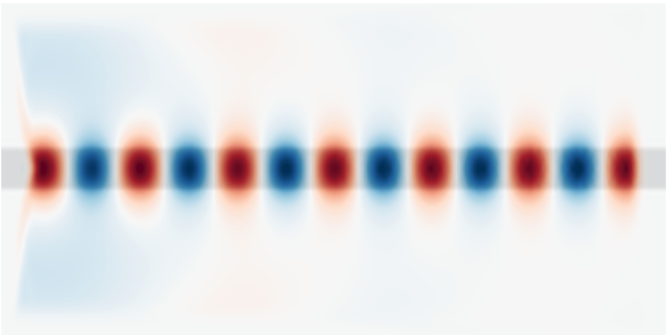We see that the the source has excited the waveguide mode but has also excited radiating fields propagating away from the waveguide. At the boundaries, the field quickly goes to zero due to the PML.

### A 90° Bend

We'll start a new simulation where we look at the fields propagating through a waveguide bend, and we'll do a couple of other things differently as well. The simulation script is in examples/bent-waveguide.py; the notebook is examples/bent-waveguide.ipynb. As usual, the first thing to do is to load the Meep library:

import meep as mp


Then let's set up the bent waveguide in a slightly larger cell:

cell = mp.Vector3(16,16,0)
geometry = [mp.Block(mp.Vector3(12,1,mp.inf),
center=mp.Vector3(-2.5,-3.5),
material=mp.Medium(epsilon=12)),
mp.Block(mp.Vector3(1,12,mp.inf),
center=mp.Vector3(3.5,2),
material=mp.Medium(epsilon=12))]
pml_layers = [mp.PML(1.0)]
resolution = 10


Note that we have two blocks, both off-center to produce the bent waveguide structure pictured below. As illustrated in the figure, the origin (0,0) of the coordinate system is at the center of the cell, with positive $y$ being downwards, and thus the block of size 12$\times$1 is centered at (-2,-3.5). Also shown in green is the source plane at $x=-7$ which is shifted to $y=-3.5$ so that it is still inside the waveguide.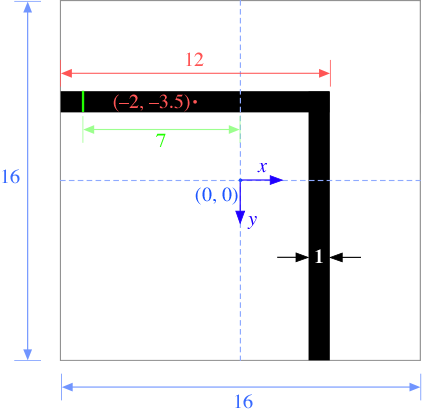There are a couple of items to note. First, a point source does not couple very efficiently to the waveguide mode, so we'll expand this into a line source, centered at (-7,-3.5), with the same width as the waveguide by adding a size property to the source. This is shown in green in the figure above. An eigenmode source can also be used which is described in Tutorial/Optical Forces. Second, instead of turning the source on suddenly at $t=0$ which excites many other frequencies because of the discontinuity, we will ramp it on slowly. Meep uses a hyperbolic tangent (tanh) turn-on function over a time proportional to the width of 20 time units which is a little over three periods. Finally, just for variety, we'll specify the vacuum wavelength instead of the frequency; again, we'll use a wavelength such that the waveguide is half a wavelength wide.

sources = [mp.Source(mp.ContinuousSource(wavelength=2*(11**0.5), width=20),
component=mp.Ez,
center=mp.Vector3(-7,-3.5),
size=mp.Vector3(0,1))]


Finally, we'll run the simulation. The first set of arguments to the run routine specify fields to output or other kinds of analyses at each time step.

sim = mp.Simulation(cell_size=cell,
boundary_layers=pml_layers,
geometry=geometry,
sources=sources,
resolution=resolution)

sim.run(mp.at_beginning(mp.output_epsilon),
mp.to_appended("ez", mp.at_every(0.6, mp.output_efield_z)),
until=200)


We are outputting the dielectric function ε but have wrapped its output function which would otherwise run at every time step in at_beginning, which does just what it says. There are several other such functions to modify the output behavior — and you can, of course, write your own, and in fact you can do any computation or output you want at any time during the time evolution and even modify the simulation while it is running.

Instead of running output_efield_z only at the end of the simulation, however, we run it at every 0.6 time units (about 10 times per period) via mp.at_every(0.6, mp.output_efield_z). By itself, this would output a separate file for every different output time, but instead we'll use another feature to output to a single 3d HDF5 file, where the third dimension is time. "ez" determines the name of the output file, which will be called ez.h5 if you are running interactively or will be prefixed with the name of the file name for a Python file (e.g. tutorial-ez.h5 for tutorial.py). If we run h5ls on this file (a standard utility, included with HDF5, that lists the contents of the HDF5 file), we get:

unix% h5ls ez.h5
ez                       Dataset {160, 160, 333/Inf}


That is, the file contains a single dataset ez that is a 160x160x333 array, where the last dimension is time. This is rather a large file, 66MB; later, we'll see ways to reduce this size if we only want images. We have a number of choices of how to output the fields. To output a single time slice, we can use the same h5topng command, but with an additional -t option to specify the time index: e.g. h5topng -t 332 will output the last time slice, similar to before. Instead, let's create an animation of the fields as a function of time. First, we have to create images for all of the time slices:

unix% h5topng -t 0:332 -R -Zc dkbluered -a yarg -A eps-000000.00.h5 ez.h5


This is similar to the command before with two new options: -t 0:332 outputs images for all time indices from 0 to 332, i.e. all of the times, and the the -R flag tells h5topng to use a consistent color scale for every image (instead of scaling each image independently). Then, we have to convert these images into an animation in some format. For this, we'll use the free ImageMagick convert program and there are other tools that work as well.

unix% convert ez.t*.png ez.gif


We are using an animated GIF format for the output. This results in the following animation:It is clear that the transmission around the bend is rather low for this frequency and structure — both large reflection and large radiation loss are clearly visible. Moreover, since we are operating just barely below the cutoff for single-mode behavior, we are able to excite a second leaky mode after the waveguide bend, whose second-order mode pattern (superimposed with the fundamental mode) is apparent in the animation. Below, we show a field snapshot from a simulation with a larger cell along the $y$ direction, in which you can see that the second-order leaky mode decays away, leaving us with the fundamental mode propagating downward.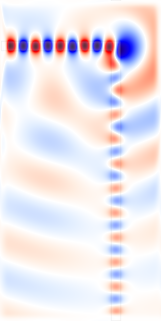Instead of doing an animation, another interesting possibility is to make an image from a $x \times t$ slice. To get the $y=-3.5$ slice, which gives us an image of the fields in the first waveguide branch as a function of time, we can use get_array in a step function to collect a slice for each time step:

vals = []

def get_slice(sim):
vals.append(sim.get_array(center=mp.Vector3(0,-3.5), size=mp.Vector3(16,0), component=mp.Ez))

sim.run(mp.at_beginning(mp.output_epsilon),
mp.at_every(0.6, get_slice),
until=200)

import matplotlib.pyplot as plt

plt.figure()
plt.imshow(vals, interpolation='spline36', cmap='RdBu')
plt.axis('off')
plt.show()#### Output Tips and Tricks

Above, we outputted the full 2d data slice at every 0.6 time units, resulting in a 69MB file. This is not large but you can imagine how big the output file would get if we were doing a 3d simulation, or even a larger 2d simulation — one can easily generate gigabytes of files, which is not only wasteful but is also slow. Instead, it is possible to output more efficiently if you know what you want to look at.

To create the movie above, all we really need are the images corresponding to each time. Images can be stored much more efficiently than raw arrays of numbers — to exploit this fact, Meep allows you to output PNG images instead of HDF5 files. In particular, instead of output_efield_z as above, we can use mp.output_png(mp.Ez, "-Zc dkbluered"), where Ez is the component to output and the "-Zc dkbluered" are options for h5topng of h5utils which is the program that is actually used to create the image files. That is:

sim.run(mp.at_every(0.6 , mp.output_png(mp.Ez, "-Zc dkbluered")), until=200)


will output a PNG file file every 0.6 time units, which can then be combined with convert as above to create a movie. The movie will be similar to the one before, but not identical because of how the color scale is determined. Before, we used the -R option to make h5topng use a uniform color scale for all images, based on the minimum/maximum field values over all time steps. That is not possible because we output an image before knowing the field values at future time steps. Thus, what output_png does is to set its color scale based on the minimum/maximum field values from all past times — therefore, the color scale will slowly "ramp up" as the source turns on.

The above command outputs zillions of PNG files, and it is somewhat annoying to have them clutter up our working directory. Instead, we can add the following command before run:

sim.use_output_directory()


This will put all of the output files (.h5, .png, etcetera) into a newly-created subdirectory, called by default filename-out/ if our Python script is filename.py.

What if we want to output an $x \times t$ slice, as above? To do this, we only really wanted the values at $y=-3.5$, and therefore we can exploit another powerful output feature — Meep allows us to output only a subset of the computational cell. This is done using the in_volume function, which like at_every and to_appended is another function that modifies the behavior of other output functions. In particular, we can do:

sim.run(mp.in_volume(mp.Volume(mp.Vector3(0,-3.5), size=mp.Vector3(16,0)), mp.to_appended("ez-slice", mp.output_efield_z)), until=200)


The first argument to in_volume is a volume which applies to all of the nested output functions. Note that to_appended, at_every, and in_volume are cumulative regardless of what order you put them in. This creates the output file ez-slice.h5 which contains a dataset of size 162x330 corresponding to the desired $x \times t$ slice.

## Transmittance Spectrum of a Waveguide Bend

We have computed the field patterns for light propagating around a waveguide bend. While this can be visually informative, the results are not quantitatively satisfying. We'd like to know exactly how much power makes it around the bend (transmittance), how much is reflected (reflectance), and how much is radiated away (scattered loss). How can we do this?

The basic principles are described in Introduction/Transmittance/Reflectance Spectra. The computation involves keeping track of the fields and their Fourier transform in a certain region, and from this computing the flux of electromagnetic energy as a function of $\omega$. Moreover, we'll get an entire spectrum of the transmittance in a single run, by Fourier-transforming the response to a short pulse. However, in order to normalize the transmitted flux by the incident power to obtain the transmittance, we'll have to do two runs, one with and one without the bend (i.e., a straight waveguide).

The simulation script is in examples/bend-flux.py. The notebook is examples/bend-flux.ipynb.

import meep as mp
import numpy as np
import matplotlib.pyplot as plt

resolution = 10 # pixels/um

sx = 16  # size of cell in X direction
sy = 32  # size of cell in Y direction
cell = mp.Vector3(sx,sy,0)

dpml = 1.0
pml_layers = [mp.PML(dpml)]


We'll also define a couple of parameters to set the width of the waveguide and the "padding" between it and the edge of the cell:

pad = 4  # padding distance between waveguide and cell edge
w = 1    # width of waveguide


In order to define the waveguide positions, we will have to use arithmetic to define the horizontal and vertical waveguide centers as:

wvg_xcen =  0.5*(sx-w-2*pad)  # x center of horiz. wvg
wvg_ycen = -0.5*(sy-w-2*pad)  # y center of vert. wvg


We proceed to define the geometry. We have to do two simulations with different geometries: the bend, and also a straight waveguide for normalization. We will first set up the straight waveguide.

geometry = [mp.Block(size=mp.Vector3(mp.inf,w,mp.inf),
center=mp.Vector3(0,wvg_ycen,0),
material=mp.Medium(epsilon=12))]


The source is a GaussianSource instead of a ContinuousSource, parameterized by a center frequency and a frequency width (the width of the Gaussian spectrum).

fcen = 0.15  # pulse center frequency
df = 0.1     # pulse width (in frequency)
sources = [mp.Source(mp.GaussianSource(fcen,fwidth=df),
component=mp.Ez,
center=mp.Vector3(-0.5*sx+dpml,wvg_ycen,0),
size=mp.Vector3(0,w,0))]


Notice how we're using our parameters like wvg_ycen and w: if we change the dimensions, everything will shift automatically.

Finally, we have to specify where we want Meep to compute the flux spectra, and at what frequencies. This must be done after specifying the Simulation object which contains the geometry, sources, resolution, etcetera, because all of the field parameters are initialized when flux planes are created. As described in Introduction/Transmittance/Reflectance Spectra, the flux is the integral of the Poynting vector over the specified FluxRegion. It only integrates one component of the Poynting vector and the direction property specifies which component. In this example, since the FluxRegion is a line, the direction is its normal by default which therefore does not need to be explicitly defined.

sim = mp.Simulation(cell_size=cell,
boundary_layers=pml_layers,
geometry=geometry,
sources=sources,
resolution=resolution)

nfreq = 100  # number of frequencies at which to compute flux

# reflected flux
refl_fr = mp.FluxRegion(center=mp.Vector3(-0.5*sx+dpml+0.5,wvg_ycen,0), size=mp.Vector3(0,2*w,0))
refl = sim.add_flux(fcen, df, nfreq, refl_fr)

# transmitted flux
tran_fr = mp.FluxRegion(center=mp.Vector3(0.5*sx-dpml,wvg_ycen,0), size=mp.Vector3(0,2*w,0))
tran = sim.add_flux(fcen, df, nfreq, tran_fr)


We compute the fluxes through a line segment twice the width of the waveguide, located at the beginning or end of the waveguide. Note that the flux lines are separated by length dpml from the boundary of the cell, so that they do not lie within the absorbing PML regions. Again, there are two cases: the transmitted flux is either computed at the right or the bottom of the cell, depending on whether the waveguide is straight or bent.

The fluxes will be computed for nfreq=100 frequencies centered on fcen, from fcen-df/2 to fcen+df/2. That is, we only compute fluxes for frequencies within our pulse bandwidth. This is important because, far outside the pulse bandwidth, the spectral power is so low that numerical errors make the computed fluxes useless.

As described in Introduction/Transmittance/Reflectance Spectra, computing the reflection spectra requires some care because we need to separate the incident and reflected fields. We do this by first saving the Fourier-transformed fields from the normalization run. And then, before we start the second run, we load these fields, negated. The latter subtracts the Fourier-transformed incident fields from the Fourier transforms of the scattered fields. Logically, we might subtract these after the run, but it turns out to be more convenient to subtract the incident fields first and then accumulate the Fourier transform. All of this is accomplished with two commands which use the raw simulation data: get_flux_data and load_minus_flux_data. We run the first simulation as follows:

pt = mp.Vector3(0.5*sx-dpml-0.5,wvg_ycen)

sim.run(until_after_sources=mp.stop_when_fields_decayed(50,mp.Ez,pt,1e-3))

# for normalization run, save flux fields data for reflection plane
straight_refl_data = sim.get_flux_data(refl)


We need to keep running after the source has turned off because we must give the pulse time to propagate completely across the cell. Moreover, the time required is a bit tricky to predict when you have complex structures, because there might be resonant phenomena that allow the source to bounce around for a long time. Therefore, it is convenient to specify the run time in a different way: instead of using a fixed time, we require that $|E_z|^2$ at the end of the waveguide must have decayed by a given amount (1/1000) from its peak value.

The stop_when_fields_decayed routine takes four arguments: dT, component, pt, and decay_by. What it does is, after the sources have turned off, it keeps running for an additional dT time units every time the given $|component|^2$ at the given point has not decayed by at least decay_by from its peak value for all times within the previous dT. In this case, dT=50, the component is $E_z$, the point is at the center of the flux plane at the end of the waveguide, and decay_by=0.001. So, it keeps running for an additional 50 time units until the square amplitude has decayed by 1/1000 from its peak. This should be sufficient to ensure that the Fourier transforms have converged.

Finally, we save the incident flux using get_fluxes which will be used later to compute the reflectance and the transmittance:

# save incident power for transmission plane
straight_tran_flux = mp.get_fluxes(tran)


We need to run the second simulation which involves the waveguide bend. We reset the structure and fields using reset_meep() and redefine the geometry, Simulation, and flux objects. At the end of the simulation, we save the reflected and transmitted fluxes.

sim.reset_meep()

sim = mp.Simulation(cell_size=cell,
boundary_layers=pml_layers,
geometry=geometry,
sources=sources,
resolution=resolution)

# reflected flux
refl = sim.add_flux(fcen, df, nfreq, refl_fr)

tran_fr = mp.FluxRegion(center=mp.Vector3(wvg_xcen,0.5*sy-dpml-0.5,0), size=mp.Vector3(2*w,0,0))
tran = sim.add_flux(fcen, df, nfreq, tran_fr)

# for normal run, load negated fields to subtract incident from refl. fields

pt = mp.Vector3(wvg_xcen,0.5*sy-dpml-0.5)

sim.run(until_after_sources=mp.stop_when_fields_decayed(50, mp.Ez, pt, 1e-3))

bend_refl_flux = mp.get_fluxes(refl)
bend_tran_flux = mp.get_fluxes(tran)

flux_freqs = mp.get_flux_freqs(refl)


With the flux data, we are ready to compute and plot the reflectance and transmittance. The reflectance is the reflected flux divided by the incident flux. We also have to multiply by -1 because all fluxes in Meep are computed in the positive-coordinate direction by default, and we want the flux in the $-x$ direction. The transmittance is the transmitted flux divided by the incident flux. Finally, the scattered loss is simply $1-transmittance-reflectance$. The results are plotted in the accompanying figure.

wl = []
Rs = []
Ts = []
for i in range(nfreq):
wl = np.append(wl, 1/flux_freqs[i])
Rs = np.append(Rs,-bend_refl_flux[i]/straight_tran_flux[i])
Ts = np.append(Ts,bend_tran_flux[i]/straight_tran_flux[i])

if mp.am_master():
plt.figure()
plt.plot(wl,Rs,'bo-',label='reflectance')
plt.plot(wl,Ts,'ro-',label='transmittance')
plt.plot(wl,1-Rs-Ts,'go-',label='loss')
plt.axis([5.0, 10.0, 0, 1])
plt.xlabel("wavelength (μm)")
plt.legend(loc="upper right")
plt.show()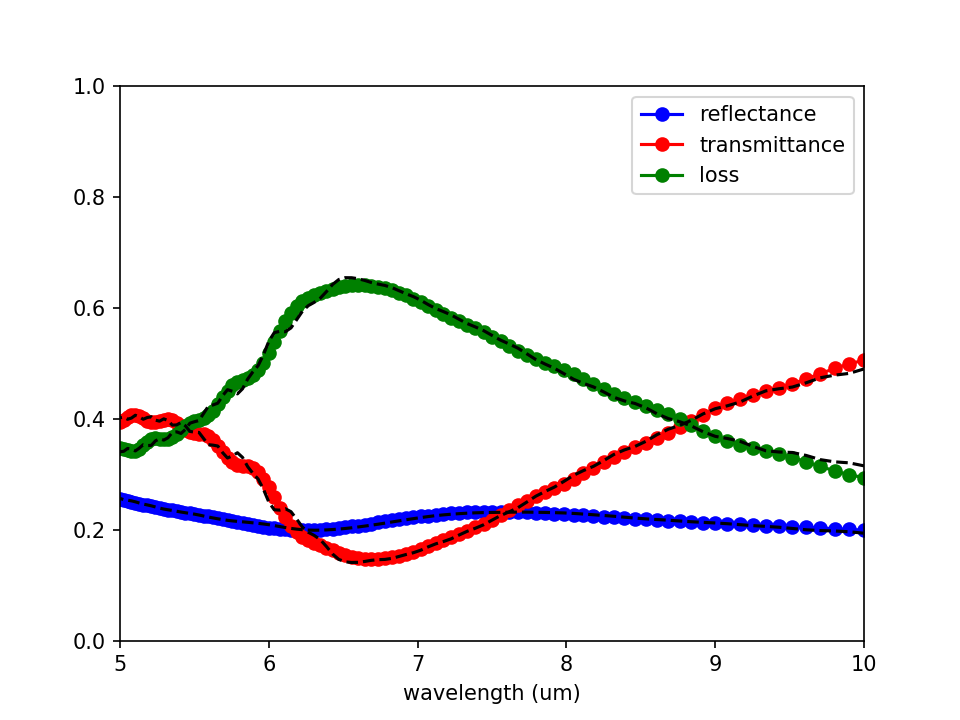We should also check whether our data is converged. We can do this by increasing the resolution and cell size and seeing by how much the numbers change. In this case, we'll try doubling the cell size:

sx=32
sy=64


Again, we must run both simulations in order to get the normalization right. The results are included in the plot above as dotted lines — you can see that the numbers have changed slightly for transmittance and loss, probably stemming from interference between light radiated directly from the source and light propagating around the waveguide.

## Angular Reflectance Spectrum of a Planar Interface

We turn to a similar but slightly different example for which there exists an analytic solution via the Fresnel equations: computing the broadband reflectance spectrum of a planar air-dielectric interface for an incident planewave over a range of angles. Similar to the previous example, we will need to run two simulations: (1) an empty cell with air/vacuum ($n=1$) everywhere to obtain the incident flux, and (2) with the dielectric ($n=3.5$) interface to obtain the reflected flux. For each angle of the incident planewave, a separate simulation is necessary.

A 1d cell must be used since a higher-dimensional cell will introduce artificial modes due to band folding. We will use a Gaussian source spanning visible wavelengths of 0.4 to 0.8 μm. Unlike a continuous-wave (CW) source, a pulsed source turns off. This enables a termination condition of when there are no fields remaining in the cell (due to absorption by the PMLs) via the run function stop_when_fields_decayed, similar to the previous example.

Creating an oblique planewave source typically requires specifying two parameters: (1) for periodic structures, the Bloch-periodic wavevector $\vec{k}$ via k_point, and (2) the source amplitude function amp_func for setting the $e^{i\vec{k} \cdot \vec{r}}$ spatial dependence ($\vec{r}$ is the position vector). Since we have a 1d cell and the source is at a single point, it is not necessary to specify the source amplitude (see this 2d example for how this is done). The magnitude of the Bloch-periodic wavevector is specified according to the dispersion relation formula for a planewave in homogeneous media with index $n$: $\omega=c|\vec{k}|/n$. As the source in this example is incident from air, $|\vec{k}|$ is simply equal to the frequency $\omega$. Note that specifying $\vec{k}$ corresponds to a single frequency. Any broadband source is therefore incident at a specified angle for only a single frequency. This is described in more detail in Section 4.5 ("Efficient Frequency-Angle Coverage") in Chapter 4 ("Electromagnetic Wave Source Conditions") of the book Advances in FDTD Computational Electrodynamics: Photonics and Nanotechnology. In this example, $\omega$ is set to the minimum frequency of the pulse to produce propagating fields at all pulse frequencies. In general, any pulse frequencies which are less than any non-zero component of $\vec{k}$ will result in evanescent fields (which carry zero power to the far field, and computationally will yield exponentially small power).

In this example, the plane of incidence which contains $\vec{k}$ and the surface normal vector is chosen to be $xz$. The source angle $\theta$ is defined in degrees in the counterclockwise (CCW) direction around the $y$ axis with 0 degrees along the $+z$ axis. In Meep, a 1d cell is defined along the $z$ direction. When $\vec{k}$ is not set, only the $E_x$ and $H_y$ field components are permitted. A non-zero $\vec{k}$ results in a 3d simulation where all field components are included and are complex valued (note that the fields are real, by default). A current source with $E_x$ polarization lies within the plane of incidence and corresponds to the convention of $\mathcal{P}$-polarization. In order to model the $\mathcal{S}$-polarization, we must use an $E_y$ source. This example involves just the $\mathcal{P}$-polarization.

The simulation script is examples/refl-angular.py. The notebook is examples/refl-angular.ipynb.

import meep as mp
import argparse
import math

def main(args):

resolution = args.res

dpml = 1.0              # PML thickness
sz = 10                 # size of cell (without PMLs)
sz = 10 + 2*dpml
cell_size = mp.Vector3(0,0,sz)
pml_layers = [mp.PML(dpml)]

wvl_min = 0.4           # min wavelength
wvl_max = 0.8           # max wavelength
fmin = 1/wvl_max        # min frequency
fmax = 1/wvl_min        # max frequency
fcen = 0.5*(fmin+fmax)  # center frequency
df = fmax-fmin          # frequency width
nfreq = 50              # number of frequency bins

# rotation angle (in degrees) of source: CCW around Y axis, 0 degrees along +Z axis

# plane of incidence is xz
k = mp.Vector3(math.sin(theta_r),0,math.cos(theta_r)).scale(fmin)

# if normal incidence, force number of dimensions to be 1
if theta_r == 0:
dimensions = 1
else:
dimensions = 3

sources = [mp.Source(mp.GaussianSource(fcen,fwidth=df), component=mp.Ex, center=mp.Vector3(0,0,-0.5*sz+dpml))]

sim = mp.Simulation(cell_size=cell_size,
boundary_layers=pml_layers,
sources=sources,
k_point=k,
dimensions=dimensions,
resolution=resolution)

refl_fr = mp.FluxRegion(center=mp.Vector3(0,0,-0.25*sz))
refl = sim.add_flux(fcen, df, nfreq, refl_fr)

sim.run(until_after_sources=mp.stop_when_fields_decayed(50, mp.Ex, mp.Vector3(0,0,-0.5*sz+dpml), 1e-9))

empty_flux = mp.get_fluxes(refl)
empty_data = sim.get_flux_data(refl)
sim.reset_meep()

# add a block with n=3.5 for the air-dielectric interface
geometry = [mp.Block(mp.Vector3(mp.inf,mp.inf,0.5*sz), center=mp.Vector3(0,0,0.25*sz), material=mp.Medium(index=3.5))]

sim = mp.Simulation(cell_size=cell_size,
geometry=geometry,
boundary_layers=pml_layers,
sources=sources,
k_point=k,
dimensions=dimensions,
resolution=resolution)

refl = sim.add_flux(fcen, df, nfreq, refl_fr)

sim.run(until_after_sources=mp.stop_when_fields_decayed(50, mp.Ex, mp.Vector3(0,0,-0.5*sz+dpml), 1e-9))

refl_flux = mp.get_fluxes(refl)
freqs = mp.get_flux_freqs(refl)

for i in range(nfreq):
print("refl:, {}, {}, {}, {}".format(k.x,1/freqs[i],math.degrees(math.asin(k.x/freqs[i])),-refl_flux[i]/empty_flux[i]))

if __name__ == '__main__':
parser = argparse.ArgumentParser()
parser.add_argument('-res', type=int, default=200, help='resolution (default: 200 pixels/um)')
parser.add_argument('-theta', type=float, default=0, help='angle of incident planewave (default: 0 degrees)')
args = parser.parse_args()
main(args)


The simulation script above computes and prints to standard output the reflectance at each frequency. Also included in the output is the wavevector component $k_x$ and the corresponding angle for the $(k_x,\omega)$ pair. For those frequencies not equal to the minimum frequency of the source, this is not the same as the specified angle of the incident planewave, but rather $\sin^{-1}(k_x/\omega)$.

Note that there are two argument parameters which can be passed to the script at runtime: the resolution of the grid and the angle of the incident planewave. The following Bash shell script runs the simulation for the angular range of 0$^\circ$ to 80$^\circ$ in increments of 5$^\circ$. For each run, the script pipes the output to one file and extracts the reflectance data to a different file.

#!/bin/bash

for i in seq 0 5 80; do
python -u refl-angular.py -theta $i |tee -a flux_t${i}.out;
grep refl: flux_t${i}.out |cut -d , -f2- > flux_t${i}.dat;
done


Two-dimensional plots of the angular reflectance spectrum based on the simulated data and the analytic Fresnel equations are generated using the Python script below. The plots are shown in the accompanying figure with four insets. The top left inset shows the simulated and analytic reflectance spectra at a wavelength of 0.6 μm. The top right inset shows the simulated reflectance spectrum as a function of the source wavelength $\lambda$ and Bloch-periodic wavevector $k_x$: $R(\lambda, k_x)$. The lower left inset is a transformation of $R(\lambda, k_x)$ into $R(\lambda, \theta)$. Note how the range of angles depends on the wavelength. For a particular angle, the reflectance is a constant for all wavelengths due to the dispersionless dielectric. The lower right inset is the analytic reflectance spectrum computed using the Fresnel equations. There is agreement between the simulated and analytic results. The Brewster's angle, where the transmittance is 1 and the reflectance is 0, is $\tan^{-1}(3.5/1)=74.1^{\circ}$. This is also verified by the simulated results.

In order to generate results for the missing portion of the reflectance spectrum (i.e., the white region), we will need to rerun the simulations for different wavelength spectra.

import matplotlib.pyplot as plt
import numpy as np
import numpy.matlib
import math

theta_in = np.arange(0,85,5)
kxs = np.empty((50,theta_in.size))
thetas = np.empty((50,theta_in.size))
Rmeep = np.empty((50,theta_in.size))

for j in range(theta_in.size):
f = np.genfromtxt("flux_t{}.dat".format(theta_in[j]), delimiter=",")
kxs[:,j] = f[:,0]
thetas[:,j] = f[:,2]
Rmeep[:,j] = f[:,3]

wvl = f[:,1]
# create a 2d matrix for the wavelength by repeating the column vector for each angle
wvls = np.matlib.repmat(np.reshape(wvl, (wvl.size,1)),1,theta_in.size)

plt.figure()
plt.pcolormesh(kxs, wvls, Rmeep, cmap='hot', shading='gouraud', vmin=0, vmax=Rmeep.max())
plt.axis([kxs[0,0], kxs[0,-1], wvl[-1], wvl])
plt.yticks([t for t in np.arange(0.4,0.9,0.1)])
plt.xlabel("Bloch-periodic wavevector ($k_x/2π$)")
plt.ylabel("wavelength (μm)")
plt.title("reflectance (meep)")
cbar = plt.colorbar()
cbar.set_ticks([t for t in np.arange(0,0.4,0.1)])
cbar.set_ticklabels(["{:.1f}".format(t) for t in np.arange(0,0.4,0.1)])
plt.show()

plt.figure()
plt.pcolormesh(thetas, wvls, Rmeep, cmap='hot', shading='gouraud', vmin=0, vmax=Rmeep.max())
plt.axis([thetas.min(), thetas.max(), wvl[-1], wvl])
plt.xticks([t for t in range(0,100,20)])
plt.yticks([t for t in np.arange(0.4,0.9,0.1)])
plt.xlabel("angle of incident planewave (degrees)")
plt.ylabel("wavelength (μm)")
plt.title("reflectance (meep)")
cbar = plt.colorbar()
cbar.set_ticks([t for t in np.arange(0,0.4,0.1)])
cbar.set_ticklabels(["{:.1f}".format(t) for t in np.arange(0,0.4,0.1)])
plt.show()

n1=1
n2=3.5

# compute angle of refracted planewave in medium n2
# for incident planewave in medium n1 at angle theta_in
theta_out = lambda theta_in: math.asin(n1*math.sin(theta_in)/n2)

# compute Fresnel reflectance for P-polarization in medium n2
# for incident planewave in medium n1 at angle theta_in
Rfresnel = lambda theta_in: math.fabs((n1*math.cos(theta_out(theta_in))-n2*math.cos(theta_in))/(n1*math.cos(theta_out(theta_in))+n2*math.cos(theta_in)))**2

Ranalytic = np.empty((50, theta_in.size))
for m in range(wvl.size):
for n in range(theta_in.size):

plt.figure()
plt.pcolormesh(thetas, wvls, Ranalytic, cmap='hot', shading='gouraud', vmin=0, vmax=Ranalytic.max())
plt.axis([thetas.min(), thetas.max(), wvl[-1], wvl])
plt.xticks([t for t in range(0,100,20)])
plt.yticks([t for t in np.arange(0.4,0.9,0.1)])
plt.xlabel("angle of incident planewave (degrees)")
plt.ylabel("wavelength (μm)")
plt.title("reflectance (analytic)")
cbar = plt.colorbar()
cbar.set_ticks([t for t in np.arange(0,0.4,0.1)])
cbar.set_ticklabels(["{:.1f}".format(t) for t in np.arange(0,0.4,0.1)])
plt.show()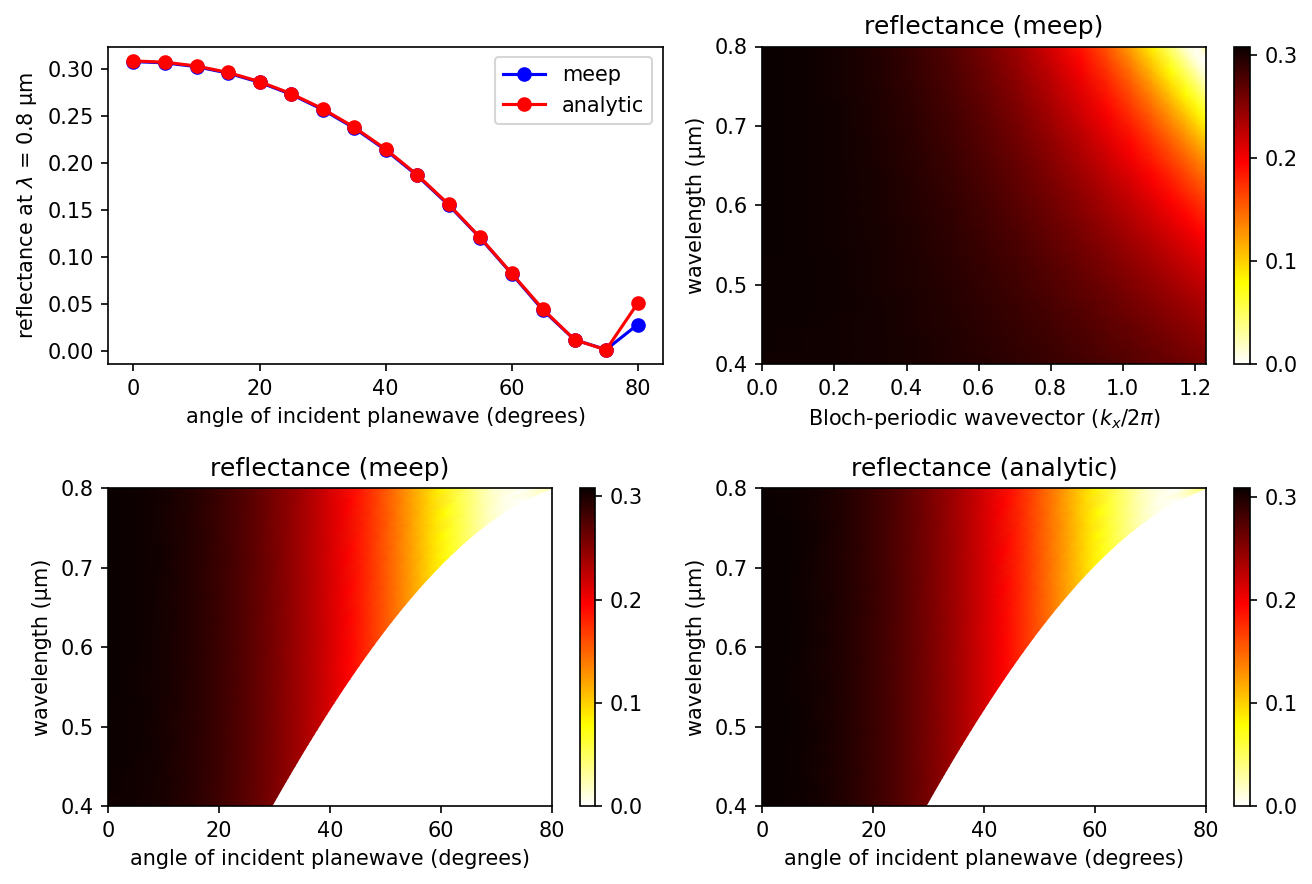## Mie Scattering of a Lossless Dielectric Sphere

A common reference calculation in computational electromagnetics for which an analytical solution is known is Mie scattering which involves computing the scattering efficiency of a single, homogeneous sphere given an incident planewave. The scattered power of any object (absorbing or non) can be computed by surrounding it with a closed DFT flux box (its size and orientation are irrelevant because of Poynting's theorem) and performing two simulations: (1) a normalization run involving an empty cell to save the incident fields from the source and (2) the scattering run with the object but first subtracting the incident fields in order to obtain just the scattered fields. This approach has already been described in Transmittance Spectrum of a Waveguide Bend.

The scattering cross section ($\sigma_{scat}$) is the scattered power in all directions divided by the incident intensity. The scattering efficiency, a dimensionless quantity, is the ratio of the scattering cross section to the cross sectional area of the sphere. In this demonstration, the sphere is a lossless dielectric with wavelength-independent refractive index of 2.0. This way, subpixel smoothing can improve accuracy at low resolutions which is important for reducing the size of this 3d simulation. The source is an $E_z$-polarized, planewave pulse (its size parameter fills the entire cell in 2d) spanning the broadband wavelength spectrum of 10% to 50% the circumference of the sphere. There is one subtlety: since the planewave source extends into the PML which surrounds the cell on all sides, is_integrated=True must be specified in the source object definition. A k_point of zero specifying periodic boundary conditions is necessary in order for the source to be infinitely extended. Also, given the symmetry of the fields and the structure, two mirror symmery planes can be used to reduce the cell size by a factor of four. The simulation results are validated by comparing with the analytic theory obtained from the PyMieScatt module (which you will have to install in order to run the script below).

A schematic of the 2d cross section at $z=0$ of the 3d cell is shown below.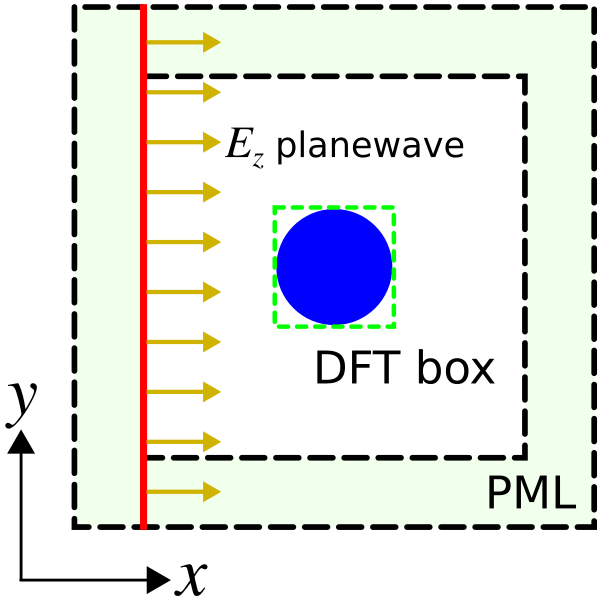The simulation script is in examples/mie_scattering.py. The notebook is examples/mie_scattering.ipynb. As an estimate of runtime, the parallel simulation on a machine with three Intel Xeon 4.20 GHz cores takes less than five minutes.

import meep as mp
import numpy as np
import matplotlib.pyplot as plt
import PyMieScatt as ps

r = 1.0  # radius of sphere

wvl_min = 2*np.pi*r/10
wvl_max = 2*np.pi*r/2

frq_min = 1/wvl_max
frq_max = 1/wvl_min
frq_cen = 0.5*(frq_min+frq_max)
dfrq = frq_max-frq_min
nfrq = 100

## at least 8 pixels per smallest wavelength, i.e. np.floor(8/wvl_min)
resolution = 25

dpml = 0.5*wvl_max
dair = 0.5*wvl_max

pml_layers = [mp.PML(thickness=dpml)]

symmetries = [mp.Mirror(mp.Y),
mp.Mirror(mp.Z,phase=-1)]

s = 2*(dpml+dair+r)
cell_size = mp.Vector3(s,s,s)

# is_integrated=True necessary for any planewave source extending into PML
sources = [mp.Source(mp.GaussianSource(frq_cen,fwidth=dfrq,is_integrated=True),
center=mp.Vector3(-0.5*s+dpml),
size=mp.Vector3(0,s,s),
component=mp.Ez)]

sim = mp.Simulation(resolution=resolution,
cell_size=cell_size,
boundary_layers=pml_layers,
sources=sources,
k_point=mp.Vector3(),
symmetries=symmetries)

box_x1 = sim.add_flux(frq_cen, dfrq, nfrq, mp.FluxRegion(center=mp.Vector3(x=-r),size=mp.Vector3(0,2*r,2*r)))
box_x2 = sim.add_flux(frq_cen, dfrq, nfrq, mp.FluxRegion(center=mp.Vector3(x=+r),size=mp.Vector3(0,2*r,2*r)))
box_y1 = sim.add_flux(frq_cen, dfrq, nfrq, mp.FluxRegion(center=mp.Vector3(y=-r),size=mp.Vector3(2*r,0,2*r)))
box_y2 = sim.add_flux(frq_cen, dfrq, nfrq, mp.FluxRegion(center=mp.Vector3(y=+r),size=mp.Vector3(2*r,0,2*r)))
box_z1 = sim.add_flux(frq_cen, dfrq, nfrq, mp.FluxRegion(center=mp.Vector3(z=-r),size=mp.Vector3(2*r,2*r,0)))
box_z2 = sim.add_flux(frq_cen, dfrq, nfrq, mp.FluxRegion(center=mp.Vector3(z=+r),size=mp.Vector3(2*r,2*r,0)))

sim.run(until_after_sources=10)

freqs = mp.get_flux_freqs(box_x1)
box_x1_data = sim.get_flux_data(box_x1)
box_x2_data = sim.get_flux_data(box_x2)
box_y1_data = sim.get_flux_data(box_y1)
box_y2_data = sim.get_flux_data(box_y2)
box_z1_data = sim.get_flux_data(box_z1)
box_z2_data = sim.get_flux_data(box_z2)

box_x1_flux0 = mp.get_fluxes(box_x1)

sim.reset_meep()

n_sphere = 2.0
geometry = [mp.Sphere(material=mp.Medium(index=n_sphere),
center=mp.Vector3(),

sim = mp.Simulation(resolution=resolution,
cell_size=cell_size,
boundary_layers=pml_layers,
sources=sources,
k_point=mp.Vector3(),
symmetries=symmetries,
geometry=geometry)

box_x1 = sim.add_flux(frq_cen, dfrq, nfrq, mp.FluxRegion(center=mp.Vector3(x=-r),size=mp.Vector3(0,2*r,2*r)))
box_x2 = sim.add_flux(frq_cen, dfrq, nfrq, mp.FluxRegion(center=mp.Vector3(x=+r),size=mp.Vector3(0,2*r,2*r)))
box_y1 = sim.add_flux(frq_cen, dfrq, nfrq, mp.FluxRegion(center=mp.Vector3(y=-r),size=mp.Vector3(2*r,0,2*r)))
box_y2 = sim.add_flux(frq_cen, dfrq, nfrq, mp.FluxRegion(center=mp.Vector3(y=+r),size=mp.Vector3(2*r,0,2*r)))
box_z1 = sim.add_flux(frq_cen, dfrq, nfrq, mp.FluxRegion(center=mp.Vector3(z=-r),size=mp.Vector3(2*r,2*r,0)))
box_z2 = sim.add_flux(frq_cen, dfrq, nfrq, mp.FluxRegion(center=mp.Vector3(z=+r),size=mp.Vector3(2*r,2*r,0)))

sim.run(until_after_sources=100)

box_x1_flux = mp.get_fluxes(box_x1)
box_x2_flux = mp.get_fluxes(box_x2)
box_y1_flux = mp.get_fluxes(box_y1)
box_y2_flux = mp.get_fluxes(box_y2)
box_z1_flux = mp.get_fluxes(box_z1)
box_z2_flux = mp.get_fluxes(box_z2)

scatt_flux = np.asarray(box_x1_flux)-np.asarray(box_x2_flux)+np.asarray(box_y1_flux)-np.asarray(box_y2_flux)+np.asarray(box_z1_flux)-np.asarray(box_z2_flux)
intensity = np.asarray(box_x1_flux0)/(2*r)**2
scatt_cross_section = np.divide(scatt_flux,intensity)
scatt_eff_meep = scatt_cross_section*-1/(np.pi*r**2)
scatt_eff_theory = [ps.MieQ(n_sphere,1000/f,2*r*1000,asDict=True)['Qsca'] for f in freqs]

if mp.am_master():
plt.figure(dpi=150)
plt.loglog(2*np.pi*r*np.asarray(freqs),scatt_eff_meep,'bo-',label='Meep')
plt.loglog(2*np.pi*r*np.asarray(freqs),scatt_eff_theory,'ro-',label='theory')
plt.grid(True,which="both",ls="-")
plt.xlabel('(sphere circumference)/wavelength, 2πr/λ')
plt.ylabel('scattering efficiency, σ/πr$^{2}$')
plt.legend(loc='upper right')
plt.title('Mie Scattering of a Lossless Dielectric Sphere')
plt.tight_layout()
plt.savefig("mie_scattering.png")


The incident intensity (intensity) is the flux in one of the six monitor planes (the one closest to and facing the planewave source propagating in the $x$ direction) divided by its area. This is why the six sides of the flux box are defined separately. (Otherwise, the entire box could have been defined as a single flux object with different weights ±1 for each side.) The scattered power is multiplied by -1 since it is the outgoing power (a positive quantity) rather than the incoming power as defined by the orientation of the flux box. Note that because of the linear $E_z$ polarization of the source, the flux through the $y$ and $z$ planes will not be the same. A circularly-polarized source would have produced equal flux in these two monitor planes. The runtime of the scattering run is chosen to be sufficiently long to ensure that the Fourier-transformed fields have converged.

Results are shown below. Overall, the Meep results agree well with the analytic theory.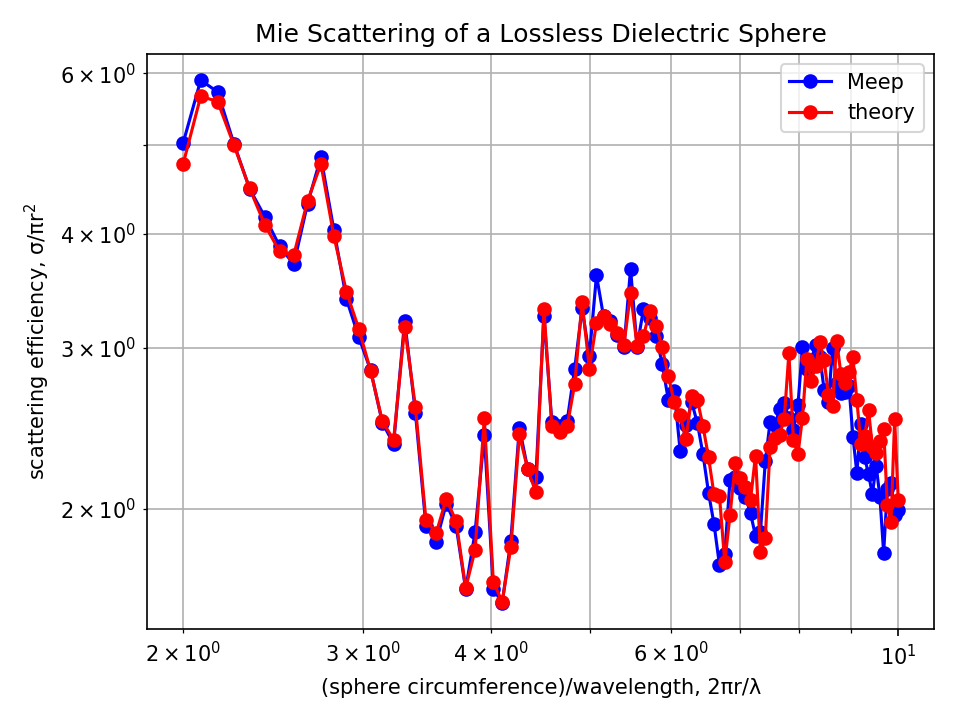Finally, for the case of a lossy dielectric material (i.e. complex refractive index) with non-zero absorption, the procedure to obtain the scattering efficiency is the same. The absorption efficiency is the ratio of the absorption cross section ($\sigma_{abs}$) to the cross sectional area of the sphere. The absorption cross section is the total absorbed power divided by the incident intensity. The absorbed power is simply flux into the same box as for the scattered power, but without subtracting the incident field (and with the opposite sign, since absorption is flux into the box and scattering is flux out of the box): omit the load_minus_flux_data calls. The extinction cross section ($\sigma_{ext}$) is simply the sum of the scattering and absorption cross sections: $\sigma_{scat}+\sigma_{abs}$.

As an extension of the Mie scattering example which involved computing the scattering cross section ($\sigma_{scat}$), we will compute the differential cross section (DCS, $\sigma_{diff}$) which is proportional to the radar cross section. Computing $\sigma_{diff}$ in a given direction involves three steps: (1) solve for the near fields on a closed box surrounding the object, (2) from the near fields, compute the far fields at a single point a large distance away (i.e., $R$ ≫ object diameter), and (3) calculate the Poynting flux of the far fields in the outward direction: $F = \hat{r}\cdot\Re[E^* \times H]$. The differential cross section in that direction is $R^2F$ divided by the incident intensity. The radar cross section (RCS) is simply $\sigma_{diff}$ in the "backwards" direction (i.e., backscattering) multiplied by $4\pi$.

The scattering cross section can be obtained by integrating the differential cross section over all spherical angles:

(In fact, this relationship is essentially the reason for the DCS definition: while the scattering cross section is total scattered power divided by incident intensity, the DCS is power per solid angle, such that integrating it over spherical angles gives the total cross section. That's why we compute DCS using the flux density in a given direction multiplied by $R^2$: in the limit $R \to \infty$, this gives the outward flux through an infinitesimal patch of an infinite sphere, divided by the solid angle of the patch. The RCS is similar, but the scattering cross section is the average of the RCS over all angles rather than the integral, which gives an additional factor of $4\pi$.)

In this demonstration, we will verify this expression for the lossless dielectric sphere at a single wavelength by comparing with the analytic theory via PyMieScatt.

The simulation script is in examples/differential_cross_section.py. The notebook is examples/differential_cross_section.ipynb.

import meep as mp
import numpy as np
import matplotlib.pyplot as plt
import PyMieScatt as ps

r = 1.0  # radius of sphere

frq_cen = 1.0

resolution = 20 # pixels/um

dpml = 0.5
dair = 1.5 # at least 0.5/frq_cen padding between source and near-field monitor

pml_layers = [mp.PML(thickness=dpml)]

s = 2*(dpml+dair+r)
cell_size = mp.Vector3(s,s,s)

# circularly-polarized source with propagation axis along x
# is_integrated=True necessary for any planewave source extending into PML
sources = [mp.Source(mp.GaussianSource(frq_cen,fwidth=0.2*frq_cen,is_integrated=True),
center=mp.Vector3(-0.5*s+dpml),
size=mp.Vector3(0,s,s),
component=mp.Ez),
mp.Source(mp.GaussianSource(frq_cen,fwidth=0.2*frq_cen,is_integrated=True),
center=mp.Vector3(-0.5*s+dpml),
size=mp.Vector3(0,s,s),
component=mp.Ey,
amplitude=1j)]

sim = mp.Simulation(resolution=resolution,
cell_size=cell_size,
boundary_layers=pml_layers,
sources=sources,
k_point=mp.Vector3())

mp.FluxRegion(center=mp.Vector3(x=-2*r),size=mp.Vector3(0,4*r,4*r)))

mp.Near2FarRegion(center=mp.Vector3(x=-2*r),size=mp.Vector3(0,4*r,4*r),weight=+1),
mp.Near2FarRegion(center=mp.Vector3(x=+2*r),size=mp.Vector3(0,4*r,4*r),weight=-1),
mp.Near2FarRegion(center=mp.Vector3(y=-2*r),size=mp.Vector3(4*r,0,4*r),weight=+1),
mp.Near2FarRegion(center=mp.Vector3(y=+2*r),size=mp.Vector3(4*r,0,4*r),weight=-1),
mp.Near2FarRegion(center=mp.Vector3(z=-2*r),size=mp.Vector3(4*r,4*r,0),weight=+1),
mp.Near2FarRegion(center=mp.Vector3(z=+2*r),size=mp.Vector3(4*r,4*r,0),weight=-1))

sim.run(until_after_sources=10)

input_flux = mp.get_fluxes(box_flux)
nearfield_box_data = sim.get_near2far_data(nearfield_box)

sim.reset_meep()

n_sphere = 2.0
geometry = [mp.Sphere(material=mp.Medium(index=n_sphere),
center=mp.Vector3(),

sim = mp.Simulation(resolution=resolution,
cell_size=cell_size,
boundary_layers=pml_layers,
sources=sources,
k_point=mp.Vector3(),
geometry=geometry)

mp.Near2FarRegion(center=mp.Vector3(x=-2*r),size=mp.Vector3(0,4*r,4*r),weight=+1),
mp.Near2FarRegion(center=mp.Vector3(x=+2*r),size=mp.Vector3(0,4*r,4*r),weight=-1),
mp.Near2FarRegion(center=mp.Vector3(y=-2*r),size=mp.Vector3(4*r,0,4*r),weight=+1),
mp.Near2FarRegion(center=mp.Vector3(y=+2*r),size=mp.Vector3(4*r,0,4*r),weight=-1),
mp.Near2FarRegion(center=mp.Vector3(z=-2*r),size=mp.Vector3(4*r,4*r,0),weight=+1),
mp.Near2FarRegion(center=mp.Vector3(z=+2*r),size=mp.Vector3(4*r,4*r,0),weight=-1))

sim.run(until_after_sources=100)

npts = 100     # number of points in [0,pi) range of polar angles to sample far fields along semi-circle
angles = np.pi/npts*np.arange(npts)

ff_r = 10000*r # radius of far-field semi-circle

E = np.zeros((npts,3),dtype=np.complex128)
H = np.zeros((npts,3),dtype=np.complex128)
for n in range(npts):
ff = sim.get_farfield(nearfield_box, ff_r*mp.Vector3(np.cos(angles[n]),0,np.sin(angles[n])))
E[n,:] = [np.conj(ff[j]) for j in range(3)]
H[n,:] = [ff[j+3] for j in range(3)]

# compute Poynting flux Pr in the radial direction.  At large r,
# all of the flux is radial so we can simply compute the magnitude of the Poynting vector.
Px = np.real(np.multiply(E[:,1],H[:,2])-np.multiply(E[:,2],H[:,1]))
Py = np.real(np.multiply(E[:,2],H[:,0])-np.multiply(E[:,0],H[:,2]))
Pz = np.real(np.multiply(E[:,0],H[:,1])-np.multiply(E[:,1],H[:,0]))
Pr = np.sqrt(np.square(Px)+np.square(Py)+np.square(Pz))

intensity = input_flux/(4*r)**2
diff_cross_section = ff_r**2 * Pr / intensity
scatt_cross_section_meep = 2*np.pi * np.sum(diff_cross_section * np.sin(angles)) * np.pi/npts # trapezoidal rule integration
scatt_cross_section_theory = ps.MieQ(n_sphere,1000/frq_cen,2*r*1000,asDict=True,asCrossSection=True)['Csca']*1e-6 # units of um^2

print("scatt:, {:.16f} (meep), {:.16f} (theory)".format(scatt_cross_section_meep,scatt_cross_section_theory))


The script is similar to the previous Mie scattering example with the main difference being the replacement of the add_flux with add_near2far objects. Instead of a linearly-polarized planewave, the source is circularly-polarized so that $\sigma_{diff}$ is invariant with the rotation angle $\phi$ around the axis of the incident direction (i.e., $x$). This way, the far fields need only be sampled with the polar angle $\theta$. A circularly-polarized planewave can be generated by overlapping two linearly-polarized planewaves ($E_y$ and $E_z$) which are 90° out of phase via specifying amplitude=1j for one of the two sources. Note, however, that there is no need to use complex fields (by specifying force_complex_fields=True in the Simulation object) which would double the floating-point memory consumption since only the real part of the source amplitude is used by default. The circularly-polarized source breaks the mirror symmetry which increases the size of the simulation. The size of the near-field monitor box surrounding the sphere is doubled so that it lies entirely within the homogeneous air region (a requirement of the near2far feature). After the near fields have been obtained for $\lambda = 1$ μm, the far fields are computed for 100 points along a semi-circle with radius 10,000X that of the dielectric sphere. (Note: any such large radius would give the same $\sigma_{scat}$ to within discretization error.) Finally, the scattered cross section is computed by numerically integrating the expression from above using the radial Poynting flux values.

The Meep results agree well with the analytic theory.

For resolution = 20, the error between the simulated and analytic result is 2.2%.

scatt:, 8.1554468215885674 (meep), 8.3429545590438750 (theory)


For resolution = 25, the error decreases (as expected) to 1.5%.

scatt:, 8.2215435272741395 (meep), 8.3429545590438750 (theory)


## Absorbed Power Density Map of a Lossy Cylinder

The dft_flux routines (add_flux) described in the previous examples compute the total power in a given region (FluxRegion). It is also possible to compute the local (i.e., position-dependent) absorbed power density in a dispersive (lossy) material. This quantity is useful for obtaining a spatial map of the photon absorption. The absorbed power density is defined as where $\mathbf{P}$ is the total polarization field. In the Fourier (frequency) domain with time-harmonic fields, this expression is where $\mathbf{E}^* \cdot \mathbf{P}$ denotes the dot product of the complex conjugate of $\mathbf{E}$ with $\mathbf{P}$. However, since $\mathbf{D}=\mathbf{E}+\mathbf{P}$, this is equivalent to since $\mathbf{E}^* \cdot \mathbf{E} = |\mathbf{E}|^2$ is purely real. Calculating this quantity involves two steps: (1) compute the Fourier-transformed $\mathbf{E}$ and $\mathbf{D}$ fields in a region via the dft_fields feature and (2) in post processing, compute $\omega\, \mathrm{Im}\, \left[ {\mathbf{E}^* \cdot \mathbf{D}} \right]$. This approach only works when the complex permittivity is specified using the Drude-Lorentzian susceptibility. Conductivity is not supported.

This tutorial example involves computing the absorbed power density for a two-dimensional cylinder (radius: 1 μm) of silicon dioxide (SiO2, from the materials library) at a wavelength of 1 μm given an incident $E_z$-polarized planewave. (The attenuation length of SiO2 at this wavelength is $\lambda/\mathrm{Im}\, \sqrt{\varepsilon}$ = ~3000 μm.) We will also verify that the total power absorbed by the cylinder obtained by integrating the absorbed power density over the entire cylinder is equivalent to the same quantity computed using the alternative method involving a closed, four-sided dft_flux box (Poynting's theorem).

The simulation script is in examples/absorbed_power_density.py. The notebook is examples/absorbed_power_density.ipynb.

import numpy as np
import matplotlib
matplotlib.use('agg')
import matplotlib.pyplot as plt

import meep as mp
from meep.materials import SiO2

resolution = 100  # pixels/um

dpml = 1.0
pml_layers = [mp.PML(thickness=dpml)]

r = 1.0     # radius of cylinder
dair = 2.0  # air padding thickness

s = 2*(dpml+dair+r)
cell_size = mp.Vector3(s,s)

wvl = 1.0
fcen = 1/wvl

# is_integrated=True necessary for any planewave source extending into PML
sources = [mp.Source(mp.GaussianSource(fcen,fwidth=0.1*fcen,is_integrated=True),
center=mp.Vector3(-0.5*s+dpml),
size=mp.Vector3(0,s),
component=mp.Ez)]

symmetries = [mp.Mirror(mp.Y)]

geometry = [mp.Cylinder(material=SiO2,
center=mp.Vector3(),
height=mp.inf)]

sim = mp.Simulation(resolution=resolution,
cell_size=cell_size,
boundary_layers=pml_layers,
sources=sources,
k_point=mp.Vector3(),
symmetries=symmetries,
geometry=geometry)

fcen,0,1,
center=mp.Vector3(),
size=mp.Vector3(2*r,2*r),
yee_grid=True)

# closed box surrounding cylinder for computing total incoming flux
mp.FluxRegion(center=mp.Vector3(x=-r),size=mp.Vector3(0,2*r),weight=+1),
mp.FluxRegion(center=mp.Vector3(x=+r),size=mp.Vector3(0,2*r),weight=-1),
mp.FluxRegion(center=mp.Vector3(y=+r),size=mp.Vector3(2*r,0),weight=-1),
mp.FluxRegion(center=mp.Vector3(y=-r),size=mp.Vector3(2*r,0),weight=+1))

sim.run(until_after_sources=100)

Dz = sim.get_dft_array(dft_fields,mp.Dz,0)
Ez = sim.get_dft_array(dft_fields,mp.Ez,0)
absorbed_power_density = 2*np.pi*fcen * np.imag(np.conj(Ez)*Dz)

dxy = 1/resolution**2
absorbed_power = np.sum(absorbed_power_density)*dxy
absorbed_flux = mp.get_fluxes(flux_box)
err = abs(absorbed_power-absorbed_flux)/absorbed_flux
print("flux:, {} (dft_fields), {} (dft_flux), {} (error)".format(absorbed_power,absorbed_flux,err))

plt.figure()
sim.plot2D()
plt.savefig('power_density_cell.png',dpi=150,bbox_inches='tight')

plt.figure()
x = np.linspace(-r,r,Dz.shape)
y = np.linspace(-r,r,Dz.shape)
plt.pcolormesh(x,
y,
np.transpose(absorbed_power_density),
cmap='inferno_r',
vmin=0,
vmax=np.amax(absorbed_power_density))
plt.xlabel("x (μm)")
plt.xticks(np.linspace(-r,r,5))
plt.ylabel("y (μm)")
plt.yticks(np.linspace(-r,r,5))
plt.gca().set_aspect('equal')
plt.title("absorbed power density" + "\n" +"SiO2 Labs(λ={} μm) = {:.2f} μm".format(wvl,wvl/np.imag(np.sqrt(SiO2.epsilon(fcen)))))
plt.colorbar()
plt.savefig('power_density_map.png',dpi=150,bbox_inches='tight')


There is one important item to note: in order to eliminate discretization artifacts when computing the $\mathbf{E}^* \cdot \mathbf{D}$ dot-product, the add_dft_fields definition includes yee_grid=True which ensures that the $E_z$ and $D_z$ fields are computed on the Yee grid rather than interpolated to the centered grid. As a corollary, we cannot use get_array_metadata to obtain the coordinates of the dft_fields region or its interpolation weights because this involves the centered grid.

A schematic of the simulation layout generated using plot2D shows the line source (red), PMLs (green hatch region), dft_flux box (solid blue contour line), and dft_fields surface (blue hatch region).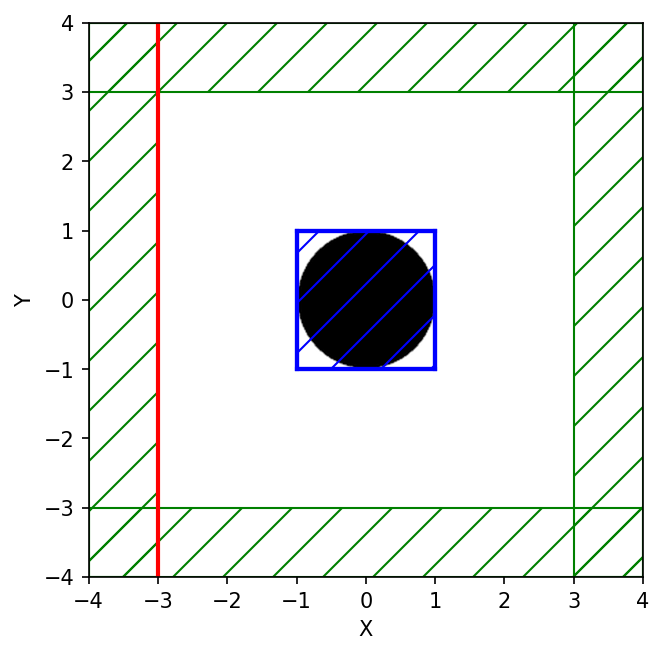The spatial map of the absorbed power density shows that most of the absorption occurs in a small region near the back surface of the cylinder (i.e., on the opposite side of the incident planewave).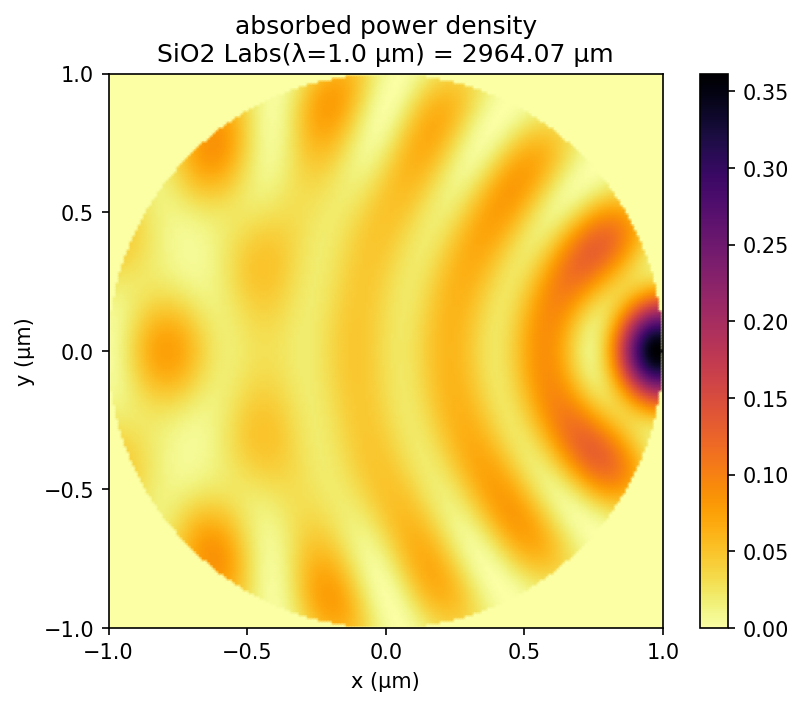Finally, the two values for the total absorbed power which are displayed at the end of the run are nearly equivalent. The relative error between the two methods is ~1.0%.

flux:, 0.13120421825956843 (dft_fields), 0.13249534167200672 (dft_flux), 0.009744670236290038 (error)


Note on units: The absorbed power density computed in this tutorial example has units of (Meep power)/(unit length)2 where (unit length) is 1 μm. To convert this quantity to a physical power for a given input power, you would multiply by (acutal power)/(Meep power flux) where (actual power) is the known physical input power and (Meep power flux) is the corresponding power meausured in Meep. For example, if you plan to have an incident planewave with a power of (actual power) = 1 mW incident on the cylinder cross-section, then you would first compute (Meep power flux) in a separate normalization run with just vacuum, measuring the DFT flux on a line segement corresponding to the cylinder diameter.

## Modes of a Ring Resonator

As described in Introduction/Resonant Modes, another common task for FDTD simulation is to find the resonant modes — frequencies and decay rates — of some cavity structure. You might want to read that again to recall the basic simulation strategy. How this works is shown in this example for a ring resonator, which is simply a waveguide bent into a circle. In fact, since this structure has cylindrical symmetry, we can simulate it much more efficiently by using cylindrical coordinates, but this demonstration involves an ordinary 2d simulation.

The simulation script is in examples/ring.py. The notebook is examples/ring.ipynb.

As before, some parameters are defined to describe the geometry, so that the structure can be easily changed:

n = 3.4                 # index of waveguide
w = 1                   # width of waveguide
r = 1                   # inner radius of ring
dpml = 2                # thickness of PML
sxy = 2*(r+w+pad+dpml)  # cell size


How do we make a circular waveguide? So far, we've only seen Block objects, but Meep also lets you specify cylinders, spheres, ellipsoids, and cones, as well as user-specified dielectric functions. In this case, we'll use two Cylinder objects, one inside the other:

c1 = mp.Cylinder(radius=r+w, material=mp.Medium(index=n))


Later objects in the geometry object take precedence over or rather lie "on top of" earlier objects, so the second air ($\varepsilon=1$) cylinder cuts a circular hole out of the larger cylinder, leaving a ring of width $w$.

We don't know the frequency of the mode(s) ahead of time, so we'll just hit the structure with a broad Gaussian pulse to excite all of the Ez-polarized modes in a chosen bandwidth:

fcen = 0.15              # pulse center frequency
df = 0.1                 # pulse frequency width
src = mp.Source(mp.GaussianSource(fcen, fwidth=df), mp.Ez, mp.Vector3(r+0.1))


Finally, we are ready to run the simulation. The basic idea is to run until the sources are finished, and then to run for some additional period of time. In that additional period, we'll perform some signal processing on the fields at some point in the cell with Harminv to identify the frequencies and decay rates of the modes that were excited:

sim = mp.Simulation(cell_size=mp.Vector3(sxy, sxy),
geometry=[c1, c2],
sources=[src],
resolution=10,
boundary_layers=[mp.PML(dpml)])

sim.run(mp.at_beginning(mp.output_epsilon),
mp.after_sources(mp.Harminv(mp.Ez, mp.Vector3(r+0.1), fcen, df)),
until_after_sources=300)


The signal processing is performed by the Harminv routine, which takes four arguments: the field component Ez and position ($r$+0.1,0) to analyze, and a frequency range given by a center frequency and bandwidth (same as the source pulse). Note that we wrap Harminv in after_sources(...), since we only want to analyze the frequencies in the source-free system (the presence of a source will distort the analysis). At the end of the run, Harminv prints a series of lines (beginning with harminv0:) listing the frequencies it found:

harminv0:, frequency, imag. freq., Q, |amp|, amplitude, error
harminv0:, 0.118101575043663, -7.31885828253851e-4, 80.683059081382, 0.00341388964904578, -0.00305022905294175-0.00153321402956404i, 1.02581433904604e-5
harminv0:, 0.147162555528154, -2.32636643253225e-4, 316.29272471914, 0.0286457663908165, 0.0193127882016469-0.0211564681361413i, 7.32532621851082e-7
harminv0:, 0.175246750722663, -5.22349801171605e-5, 1677.48461212767, 0.00721133215656089, -8.12770506086109e-4-0.00716538314235085i, 1.82066436470489e-7


There are six, comma-delimited columns in addition to the label. These results are also stored in Harminv.modes. The meaning of these columns is as follows. Harminv analyzes the fields $f(t)$ at the given point, and expresses this as a sum of modes in the specified bandwidth:

for complex amplitudes $a_n$ and complex frequencies ω$_n$. The six columns relate to these quantities. The first column is the real part of ω$_n$, expressed in our usual 2πc units, and the second column is the imaginary part — a negative imaginary part corresponds to an exponential decay. This decay rate, for a cavity, is more often expressed as a dimensionless "lifetime" $Q$, defined by:

$Q$ is the number of optical periods for the energy to decay by $\exp(-2\pi)$, and 1/$Q$ is the fractional bandwidth at half-maximum of the resonance peak in Fourier domain. This $Q$ is the third column of the output. The fourth and fifth columns are the absolute value $|a_n|$ and complex amplitudes $a_n$. The last column is a crude measure of the error in the frequency (both real and imaginary). If the error is much larger than the imaginary part, for example, then you can't trust the $Q$ to be accurate. Note: this error is only the uncertainty in the signal processing, and tells you nothing about the errors from finite resolution, finite cell size, and so on.

An interesting question is how long should we run the simulation, after the sources are turned off, in order to analyze the frequencies. With traditional Fourier analysis, the time would be proportional to the frequency resolution required, but with Harminv the time is much shorter. For example, there are three modes. The last has a $Q$ of 1677, which means that the mode decays for about 2000 periods or about 2000/0.175 = 104 time units. We have only analyzed it for about 300 time units, however, and the estimated uncertainty in the frequency is 10-7 (with an actual error of about 10-6, from below). In general, you need to increase the run time to get more accuracy, and to find very high $Q$ values, but not by much. In some cases, modes with $Q$ of around 109 can be found with only 200 periods.

In this case, we found three modes in the specified bandwidth, at frequencies of 0.118, 0.147, and 0.175, with corresponding $Q$ values of 81, 316, and 1677. As was shown by Marcatilli in 1969, the $Q$ of a ring resonator increases exponentially with the product of $\omega$ and ring radius. Suppose that we want to actually see the field patterns of these modes. No problem: we just re-run the simulation with a narrow-band source around each mode and output the field at the end.

In particular, to output the field at the end we might add an at_end(mp.output_efield_z) argument to our run_after_sources routine, but this is problematic: we might be unlucky and output at a time when the Ez field is almost zero (i.e. when all of the energy is in the magnetic field), in which case the picture will be deceptive. Instead, at the end of the run we'll output 20 field snapshots over a whole period 1/fcen by appending the command:

sim.run(mp.at_every(1/fcen/20, mp.output_efield_z), until=1/fcen)


We can get our modes just by changing two parameters and re-running:

fcen=0.118
df=0.01


After each one of these commands, we'll convert the fields into PNG images and thence into an animated GIF (as with the bend movie, above), via:

unix% h5topng -RZc dkbluered -C ring-eps-000000.00.h5 ring-ez-*.h5
unix% convert ring-ez-*.png ring-ez-0.118.gif


The resulting animations for (from left to right) 0.118, 0.147, and 0.175, are below, in which you can clearly see the radiating fields that produce the losses: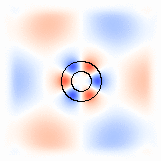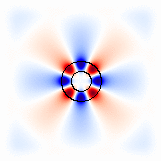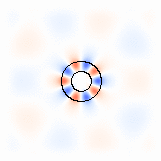Each of these modes is, of course, doubly-degenerate according to the representations of the $C_{\infty\mathrm{v}}$ symmetry group. The other mode is simply a slight rotation of this mode to make it odd through the $x$ axis, whereas we excited only the even modes due to our source symmetry. Equivalently, one can form clockwise and counter-clockwise propagating modes by taking linear combinations of the even/odd modes, corresponding to an angular $\phi$ dependence $e^{\pm i m\phi}$ for m=3, 4, and 5 in this case.

You may have noticed, by the way, that when you run with the narrow-bandwidth source, Harminv gives you slightly different frequency and $Q$ estimates, with a much smaller error estimate — this is not too strange, since by exciting a single mode you generate a cleaner signal that can be analyzed more accurately. For example, the narrow-bandwidth source for the $\omega=0.175$ mode gives:

harminv0:, 0.175247426698716, -5.20844416909221e-5, 1682.33949533974, 0.185515412838043, 0.127625313330642-0.13463932485617i, 7.35320734698267e-12


which differs by about 10-6 from the earlier estimate; the difference in $Q$ is, of course, larger because a small absolute error in ω gives a larger relative error in the small imaginary frequency.

For a demonstration of how to compute the gradient of the resonant frequency with respect to the ring radius, see Tutorial/Cylindrical Coordinates/Sensitivity Analysis via Perturbation Theory.

### Exploiting Symmetry

In this case, because we have a mirror symmetry plane (the $x$ axis) that preserves both the structure and the sources, we can exploit this mirror symmetry to speed up the computation. See also Exploiting Symmetry. In particular, everything about the script is the same except that we specify an additional object for the Simulation class:

symmetries=[mp.Mirror(mp.Y)]


This tells Meep to exploit a mirror-symmetry plane through the origin perpendicular to the $y$ direction. Meep does not check whether your system really has this symmetry — you should only specify symmetries that really preserve your structure and your sources.

Everything else about your simulation is the same: you can still get the fields at any point, the output file still covers the whole ring, and the harminv outputs are exactly the same. Internally, however, Meep is only doing computations with half of the structure, and the simulation is around twice as fast.

In general, the symmetry of the sources may require some phase. For example, if our source was in the $y$ direction instead of the $z$ direction, then the source would be odd under mirror flips through the $x$ axis. We would specify this by mp.Mirror(mp.Y, phase=-1). See Python Interface/Symmetry for more symmetry possibilities.

In this case, we actually have a lot more symmetry that we could potentially exploit, if we are willing to restrict the symmetry of our source/fields to a particular angular momentum (i.e. angular dependence $e^{im\phi}$). See also Tutorial/Cylindrical Coordinates/Modes of a Ring Resonator for how to solve for modes of this cylindrical geometry much more efficiently.

## Visualizing 3d Structures

The previous examples were based on a 1d or 2d cell in which the structure and fields can be visualized using the plotting routines in Matplotlib. In order to visualize 3d structures, you can use Mayavi. The following example involves a hexagonal prism with index 3.5 perforated by a conical hole. There are no other simulation parameters specified. The permittivity data is visualized using an isosurface plot via the contour3d module. (This functionality is automated by the plot3D routine.) A snapshot of this plot is shown below. For visualization of the vector fields in 3d, you can use the quiver3d module.

import meep as mp
import numpy as np
import math

cell_size = mp.Vector3(2,2,2)

# A hexagon is defined as a prism with six vertices centered on the origin
vertices = [mp.Vector3(-1,0),
mp.Vector3(-0.5,math.sqrt(3)/2),
mp.Vector3(0.5,math.sqrt(3)/2),
mp.Vector3(1,0),
mp.Vector3(0.5,-math.sqrt(3)/2),
mp.Vector3(-0.5,-math.sqrt(3)/2)]

geometry = [mp.Prism(vertices, height=1.0, material=mp.Medium(index=3.5)),

sim = mp.Simulation(resolution=50,
cell_size=cell_size,
geometry=geometry)

sim.init_sim()

eps_data = sim.get_epsilon()

from mayavi import mlab
s = mlab.contour3d(eps_data, colormap="YlGnBu")
mlab.show()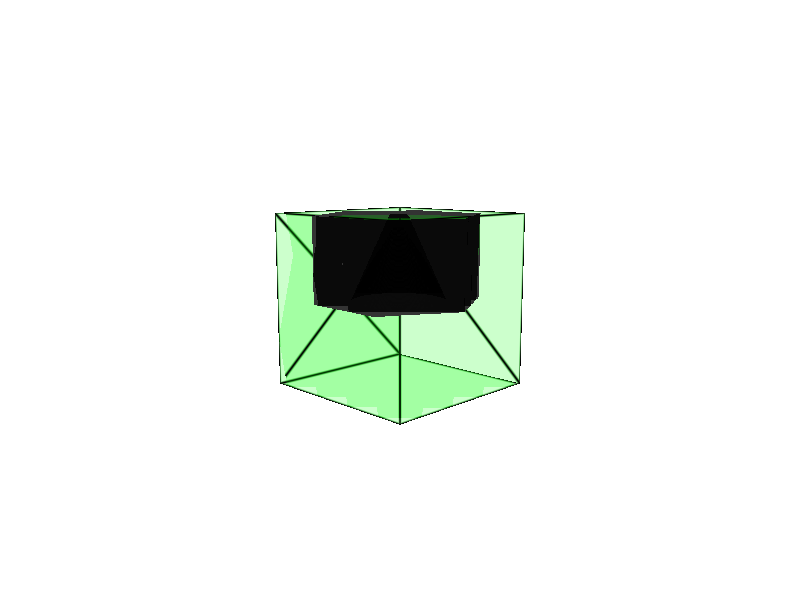Alternatively, the permittivity can be visualized from outside of Python. This involves writing the permittivity data to an HDF5 file using output_epsilon. The HDF5 data is then converted to VTK via h5tovtk of the h5utils package. VTK data can be visualized using Mayavi or Paraview.